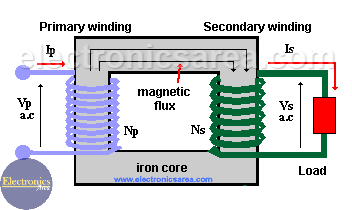# What is an ideal Transformer?

The ideal transformer a is an electromagnetic device that allows us to increase or decrease the alternating voltage and current while maintaining constant power. (Power at the output of the ideal transformer is equal to the power at its input).

## Power relationship between primary winding and secondary winding

As a consequence of the voltage transformation, there is a transformation in the electric current (alternating current) between the input and the output of the transformer, in a way that the power delivered by the transformer is the same power it receives.

On the ideal transformer:  Output Power = Input Power (PS = PP).

So, if a voltage increases, the electric current decreases in the same factor, maintaining the equality. Look at the formula.  P = VxI, then: VP x IP = VS x IS.The transformer consists of an iron core on which several sets of turns of a conductor wire have been wrapped. This set of turns are the primary (P) and the secondary (S).

## How the ideal transformer works

1. The Primary receives at its inputs, a changing voltage causing a changing electric current that flows through it.
2. The changing current will induce a magnetic flux in the iron core.
3. As the secondary winding is rolled up on the same iron core of the primary winding, the magnetic flux flows through it.
4. The magnetic flux through the coils of the “secondary” will generate a changing voltage at its outputs.
5. If there is a load connected to the outputs of the secondary, a changing electric current will flow through it (For example: the secondary winding is connected to a resistor).

## Voltage Transformation ratio

The output voltage (secondary) Vs is scaled from the input (primary) Vp, by a factor equal to the ratio of the number of turns of wire in their respective winding.   Vs/Vp = Ns/Np.

Then the output voltage at the the secondary winding is: Vs = (Ns/Np) x Vp.

Notice that the output voltage Vs, depends on the ratio of Ns (number of turns of the secondary) and Np (number of turns of the primary). Vs can be bigger or smaller than Vp.

## Current Transformation ratio

Like the voltage, the input current (Ip) is scaled from the output current (Is), by a factor equal to the radio of the numbers of turns of the wire in their respective winding. Ip / Is = Ns / Np.

If we know the electric current in the secondary winding (Is), we can find the current on the primary winding (Ip) using the following formula: Ip = (Ns/Np) x Is.

•
•
•
•
•
•
•
•
•
•
•
•
•
•
•
•
•
•